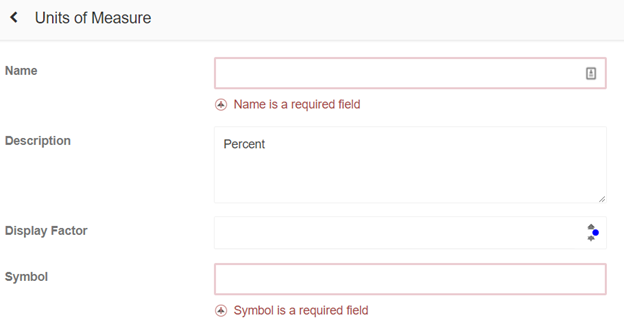Units of measure (UoM) is the measurement used and linked to a KPI.

Click the Add button to create a unit of measure. A window will open which will allow the capture of information.Name: Name is required as this is used to identify the UoM throughout the system.

Display Factor: Display factor field is used when converting the Unit to a value. If the unit of measure is a percentage < % >, enter 100 in the Factor field. This allows values to be captured/viewed as 68% instead of 0.68.

Symbol: The symbol is required as well as this is used to identify the UoM on the value.

For example, if the UoM is Ton per blast then the name would be Ton per blast, Symbol T/Blast and Display factor would be the cost of 1 ton per blast to give it a value, if the value is 100 then this should be the value therefore on 5 t/blast would equate to a value of 500.